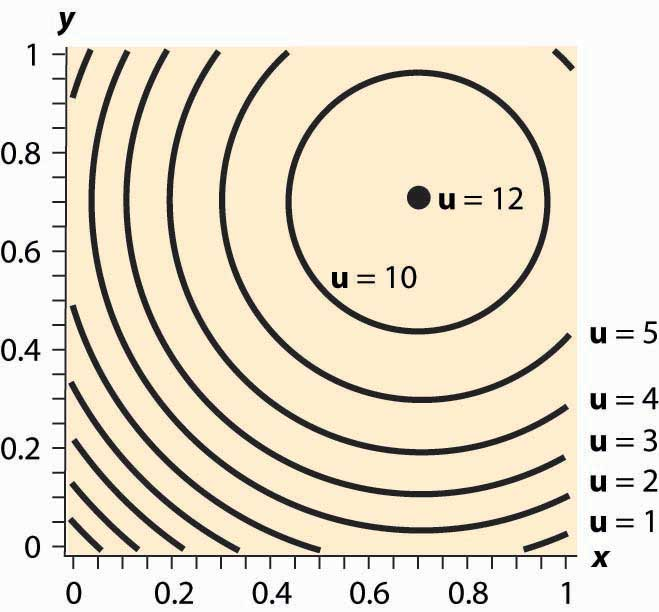# 12.4: Examples

•• Anonymous
• LibreTexts
$$\newcommand{\vecs}{\overset { \rightharpoonup} {\mathbf{#1}} }$$ $$\newcommand{\vecd}{\overset{-\!-\!\rightharpoonup}{\vphantom{a}\smash {#1}}}$$$$\newcommand{\id}{\mathrm{id}}$$ $$\newcommand{\Span}{\mathrm{span}}$$ $$\newcommand{\kernel}{\mathrm{null}\,}$$ $$\newcommand{\range}{\mathrm{range}\,}$$ $$\newcommand{\RealPart}{\mathrm{Re}}$$ $$\newcommand{\ImaginaryPart}{\mathrm{Im}}$$ $$\newcommand{\Argument}{\mathrm{Arg}}$$ $$\newcommand{\norm}{\| #1 \|}$$ $$\newcommand{\inner}{\langle #1, #2 \rangle}$$ $$\newcommand{\Span}{\mathrm{span}}$$ $$\newcommand{\id}{\mathrm{id}}$$ $$\newcommand{\Span}{\mathrm{span}}$$ $$\newcommand{\kernel}{\mathrm{null}\,}$$ $$\newcommand{\range}{\mathrm{range}\,}$$ $$\newcommand{\RealPart}{\mathrm{Re}}$$ $$\newcommand{\ImaginaryPart}{\mathrm{Im}}$$ $$\newcommand{\Argument}{\mathrm{Arg}}$$ $$\newcommand{\norm}{\| #1 \|}$$ $$\newcommand{\inner}{\langle #1, #2 \rangle}$$ $$\newcommand{\Span}{\mathrm{span}}$$$$\newcommand{\AA}{\unicode[.8,0]{x212B}}$$

##### Learning Objectives
• Are there any convenient functional forms for analyzing consumer choice?

The Cobb-Douglas utility function comes in the form u( x,y )= x α y 1−α . Since utility is zero if either of the goods is zero, we see that a consumer with Cobb-Douglas preferences will always buy some of each good. The marginal rate of substitution for Cobb-Douglas utility is

\begin{equation}-d y d x | u=u 0=\partial u \partial x \partial u \partial y=a y(1-a) x\end{equation}

Thus, the consumer’s utility maximization problem yields

\begin{equation}p X p Y=-d y d x | u=u 0=\partial u \partial x \partial u \partial y=a y(1-a) x\end{equation}

Thus, using the budget constraint, $$\begin{equation}(1-a) \times p X=a y p Y=a(M-x p X)\end{equation}$$ This yields

\begin{equation}\mathrm{x}=\mathrm{aMp} \mathrm{X}, \quad \mathrm{y}=(1-\mathrm{a}) \mathrm{M} \mathrm{p} \mathrm{Y}\end{equation}

The Cobb-Douglas utility results in constant expenditure shares. No matter what the price of X or Y, the expenditure xpX on X is αM. Similarly, the expenditure on Y is (1 – α)M. This makes the Cobb-Douglas utility very useful for computing examples and homework exercises.

When two goods are perfect complements, they are consumed proportionately. The utility that gives rise to perfect complements is in the form $$\begin{equation}u(x, y)=\min \{x, \beta y\}\end{equation}$$ for some constant β (the Greek letter “beta”). First observe that, with perfect complements, consumers will buy in such a way that x = βy. The reason is that, if x > βy, some expenditure on x is a waste since it brings in no additional utility; and the consumer gets higher utility by decreasing x and increasing y. This lets us define a “composite good” that involves buying some amount y of Y and also buying βy of X. The price of this composite commodity is $$\begin{equation}\beta \mathrm{p} \mathrm{X}+\mathrm{pY}\end{equation}$$, and it produces utility $$\begin{equation}u=M \beta p X+p Y\end{equation}$$. In this way, perfect complements boil down to a single good problem.

If the only two goods available in the world were pizza and beer, it is likely that satiation—the point at which increased consumption does not increase utility—would set in at some point. How many pizzas can you eat per month? How much beer can you drink? (Don’t answer that.)

Figure 12.8 Isoquants for a bliss pointFigure 12.8. Near the origin, the isoquants behave as before. However, as one gets full of pizza and beer, a point of maximum value is reached, illustrated by a large black dot. What does satiation mean for the theory? First, if the bliss point isn’t within reach, the theory behaves as before. With a bliss point within reach, consumption will stop at the bliss point. A feasible bliss point entails having a zero value of money. There may be people with a zero value of money, but even very wealthy people, who reach satiation in goods that they personally consume, often like to do other things with the wealth and appear not to have reached satiation overall.

## Key Takeaways

• The Cobb-Douglas utility results in constant expenditure shares.
• When two goods are perfect complements, they are consumed proportionately. Perfect complements boil down to a single good problem.
• A bliss point, or satiation, is a point at which further increases in consumption reduce utility.

## EXERCISES

1. Consider a consumer with utility $$\begin{equation}u(x, y)=x y\end{equation}$$. If the consumer has $100 to spend, and the price of X is$5 and the price of Y is \$2, graph the budget line; and then find the point that maximizes the consumer’s utility given the budget. Draw the utility isoquant through this point. What are the expenditure shares?
2. Consider a consumer with utility $$\begin{equation}u(x, y)=x y\end{equation}$$. Calculate the slope of the isoquant directly by solving u(x,y)= u 0 for y as a function of x and the utility level u0. What is the slope $$\begin{equation}-d y d x | u=u 0\end{equation}$$ ? Verify that it satisfies the formula given above.
3. Consider a consumer with utility $$\begin{equation}u(x, y)=(x y) 2\end{equation}$$. Calculate the slope of the isoquant directly by solving u(x,y)= u 0 for y as a function of x and the utility level u0. What is the slope − dy dx | u= u 0 ? Verify that the result is the same as in the previous exercise. Why is it the same?
4. The case of perfect substitutes arises when all that matters to the consumer is the sum of the products—for example, red shirts and green shirts for a colorblind consumer. In this case, $$\begin{equation}u(x, y)=x+y\end{equation}$$. Graph the isoquants for perfect substitutes. Show that the consumer maximizes utility by spending his or her entire income on whichever product is cheaper.
5. Suppose u( x,y )= x α + y α for α < 1. Show that $$\begin{equation}x=M p X(1+(p Y p X) a)\end{equation}$$ and $$\begin{equation}y=M p Y(1+(p X p Y) a)\end{equation}$$.
6. Suppose that one consumer has the utility function u (which is always a positive number), and a second consumer has utility w. Suppose, in addition, that for any x, y, $$\begin{equation}w(x, y)=(u(x, y))^{2}\end{equation}$$; that is, the second person’s utility is the square of the first person’s. Show that these consumers make the same choices—that is, $$\begin{equation}\left.u \geq x_{a}, y_{a}\right) \geq u\left(x_{b}, y_{b}\right)\end{equation}$$ if and only if $$\begin{equation}w\left(x_{a}, y_{a}\right) \geq w\left(x_{b}, y_{b}\right)\end{equation}$$

This page titled 12.4: Examples is shared under a CC BY-NC-SA 3.0 license and was authored, remixed, and/or curated by Anonymous via source content that was edited to the style and standards of the LibreTexts platform; a detailed edit history is available upon request.